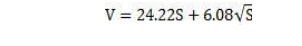Home | | High Voltage Engineering | Uniform Field Spark Gaps - Measurement of High Voltage and Currents

# Uniform Field Spark Gaps - Measurement of High Voltage and Currents

Bruce suggested the use of uniform field spark gaps for the measurements of a.c., d.c and impulse voltages. These gaps provide accuracy to within 0.2% for a.c. voltage measurements an appreciable improvement as compared with the equivalent sphere gap arrangement.

Uniform Field Spark Gaps

Bruce suggested the use of uniform field spark gaps for the measurements of a.c., d.c and impulse voltages. These gaps provide accuracy to within 0.2% for a.c. voltage measurements an appreciable improvement as compared with the equivalent sphere gap arrangement.

Fig. 4.1 shows a half-contour of one electrode having plane sparking surfaces with edges of gradually increasing curvatureThe portion AB is flat, the total diameter of the flat portion being greater than the maximum spacing between the electrodes. The portion BC consists of a sine curve based on the axes OB and OC and given by XY = CO sin (BX/BO . π/2). CD is an arc of a circle with centre at O.Bruce showed that the breakdown voltage V of a gap of length S cms in air at 20°C and 760 mmHg. Pressure is within 0.2 per cent of the value given by the empirical relation.This is a great advantage, that is, if the spacing between the spheres for breakdown is known the breakdown voltage can be calculated.

The other advantages of uniform field spark gaps are

(i)    No influence of nearby earthed objects

(ii) No polarity effect.

(i)    Very accurate mechanical finish of the electrode is required.

(ii) Careful parallel alignment of the two electrodes.

(iii)   Influence of dust brings in erratic breakdown of the gap.

This is much more serious in these gaps as compared with sphere gaps as the highly stressed electrode areas become much larger. Therefore, a uniform field gap is normally not used for voltage measurements.

Study Material, Lecturing Notes, Assignment, Reference, Wiki description explanation, brief detail
High Voltage Engineering : Measurement of High Voltage and High Currents : Uniform Field Spark Gaps - Measurement of High Voltage and Currents |